Infinite Series

An infinite series is an expression such as: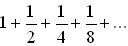where the dots imply that an infinite number of terms will be added.

 Partial Sums: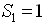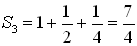and so on.
To find the sum of an infinite series, we exam the partial sums.  The sum of the series will be the limit of the partial sums (that is, the number that the partial sums are approaching, if, in fact, they do approach a number).
If the limit exists, the series is said to converge.
If the limit does not exist the series is said to diverge.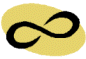The sum of an infinite series is the limit of the partial sums.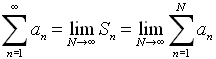On the graphing calculator:

 Method 1:

An infinite series can be examined as you would examine a finite series.  Utilize the sum feature and the seq features.  This time, however, we will "choose" an ending value for the summation.  We are looking for an idea of where the sum is heading.  Let's examine our first series:where the pattern is•  Enter the series as we did finite series. •  Examine 5 partial sums. •  Recall the entry and examine 50 partial sums. •  The limit approaches 2. Choose whichever numbers you wish.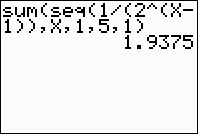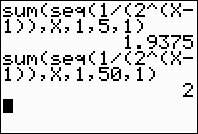Method 2:

An infinite series can also be examined using lists.   Let's examine our first series again:where the pattern isEnter the number of terms you wish to examine in L1.  Enter the formula into L2.  Enter the cumulative sum into L3.  cumSum is found 2nd LIST - OPS - #6 cumSum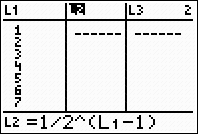Now, examine what is happening to the partial sums in L3.
Increase the number of entries if needed for further examination.

 Example:

Evaluate:Method 1:  Using sum and seq with 10 partial sums.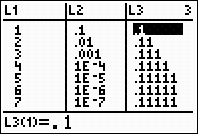Method 2:  Using the list with 10 partial sums Examining the partial sums shows that the limit is approaching .1111111111 which is 1/9.